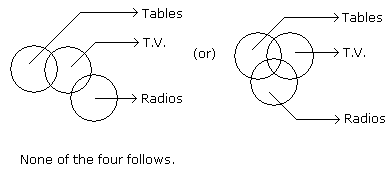# Verbal Reasoning - Syllogism - Discussion

### Discussion :: Syllogism - Syllogism 2 (Q.No.5)

In each of the following questions two statements are given. Which are followed by four conclusions (1), (2), (3) and (4). Choose the conclusions which logically follow from the given statements.

5.

Statements: Some tables are T.V. Some T.V. are radios.

Conclusions:

3. All the radios are T.V.
4. All the T.V. are tables.

 [A]. Only (2) and (4) [B]. Only (1) and (3) [C]. Only (4) [D]. Only (1) and (4) [E]. None of the four.

Explanation:Sabir said: (Feb 22, 2012) Please explain which figure we have to use to deduce result. Tell me the importance of these two figures. ? According to figure 1- answer is (E) and according to figure 2 answer is different.

 Payal said: (May 23, 2012) I don't understand this question how come E is the answer. Please explain it.

 Kumaravel said: (Sep 23, 2012) Draw Venn diagram for the Statements Provided then you can easily arrive at the solution.

 Priya said: (Apr 12, 2013) Whenever you get in question some as the starting word in both the statements, then answer should be none.

 Rajkasam said: (Oct 15, 2014) Please let me know which is correct answer, and which diagram must be used to decide the answer. According to 1st diagram option D is correct but according to 2nd diagram option varies.

 Harry said: (Nov 4, 2014) If we consider 2 diagram, it satisfies all the four options. Then why is 'none of the above' correct answer?

 Ramesh said: (Mar 31, 2015) @Sabir and @Harry are correct. If we solve with second diagram the total answer changes. If so what is the use the second one? else if second diagram holds the value? Then how come (E) will be the answer? What about first diagram then?

 Saurabh said: (Apr 24, 2015) It mean we will have to use the diagram as per options.

 Meera said: (Jun 19, 2015) Why not we consider second diagram meet?

 Bala said: (Oct 27, 2015) Answer is correct. According to figure 2 we get conclusion 1 & 2 follows but its not in option (only 1 & 2 follows). So none of the four is correct.

 Aaushi said: (May 7, 2016) In the above question, it is not compulsory that the remaining tables are radios. It can be something else. In the same way, it is not compulsory that the remaining radios are tables. So 1 and 2 not possible. And in 3 & 4 are talking about all radio and TV, whereas in question it's only about few. So E is the correct option.

 Priya said: (Jun 10, 2016) I agree with you @Bala. Option 1 & 2 follows.

 Shini said: (Aug 26, 2016) Other than Venn diagram there is another method also 100 50 method if we solve according to that method than option E is correct.

 Akash Swaminathan said: (Sep 2, 2016) Correct answer should be both (1) and (2).

 Sanjay said: (Oct 1, 2016) For the same question stated above what if they had given an option as ("only 1 and 2") and also the option ("None of These ") what would have been the answer then?

 Faithful said: (Dec 16, 2016) @Sanjay. In that case, the option of only 1 and 2 will be the correct answer. The best way to approach this is to apply the Venn Diagram Method. I totally agree with your answer @Bala.

 Siri said: (Jan 6, 2017) I didn't understand this problem. Please tell me the answer with the clear explanation.

 Siri said: (Jan 6, 2017) I didn't understand this problem. Please tell me the answer with the clear explanation.

 Siri said: (Jan 6, 2017) I didn't understand this problem. Please tell me the answer with the clear explanation.

 Ranveer said: (Feb 19, 2017) Hi Friends, When there is no direct relationship given between two statements then the following conclusion is always wrong. In this question, there is no direct relationship given between radio and table and hence conclusions 1 and 2 are false, 3 and 4th are very straight forward. However, you may think that there is a possibility that few radios are the table and vice versa but in that case word possible should be in conclusion (like is it possible that few tables can be radios) normally it doesn't have so I don't think you should take it as the true statement. Thanks.

 Abhi said: (Jun 1, 2017) According to me, the answer is 1, 3, 4.

 Bubu said: (Sep 16, 2017) No, according to me, the answer is 1, 2.

 Sruthi said: (Oct 5, 2017) @Ranveer. From what you have said I understood how the first two conclusions were wrong but I don't understand about 3 and 4 can you please explain.

 Anubhav said: (Feb 27, 2018) We have to choose the diagram with minimum intersection. That is the first one.

 Rohit said: (Mar 3, 2018) Why we have to choose the diagram with minimum intersection? please explain.

 Madhavi said: (Mar 4, 2018) Answer 1 & 2 but why the answer is 'e'? tell me, didn't understand.

 Ashwini said: (Sep 22, 2018) Option 1, 2 follows.

 Swarna Latha S said: (Dec 19, 2018) I think the conclusion 1 & 2 is correct.

 Paimon Dkhar said: (Feb 14, 2019) According to me 1, 3, 4 is correct.